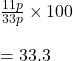## by selling 33m of cloth ,prabha gained the selling price 11 m.what is the gain percent​

Question

by selling 33m of cloth ,prabha gained the selling price 11 m.what is the gain percent​

in progress 0
6 months 2021-08-15T14:03:39+00:00 1 Answers 6 views 0

## Answers ( )

The gain percent is 33.3 %

Step-by-step explanation:

Let the selling price of 1 m cloth is p.

Cost of 33 m = 33 p

gain = cost of 11 m = 11 p

The gain percentage is given byThe gain percent is 33.3 %.